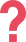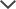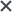## Free Sample Questions

### Verbal Reasoning Battery

The following question is a Verbal Classification question. Verbal classification questions introduce three words that share a certain connection. You must choose the word from the answer choices that share the same connection.

A. See
B. Bike
C. Abilities
D. Feel
E. Dream

Read, Calculate, and Swim are all learned abilities. Bike is also an ability that needs to be learned in order for one to have it.

See is incorrect as people do not need to learn how to see, for it is an innate ability.
Abilities is incorrect as this is the name of the general category to which read, calculate, and swim belong.
Feel is incorrect as people do not need to learn how to feel, for this is an innate ability.
Dream is incorrect as we cannot learn how to dream, for people dream in their sleep involuntarily.TestPrep-Online Developer's Tip: A good strategy to solve Verbal Classification questions is to try phrasing a sentence that fits all the given words, such as “All words can/are           ”. Then, go through the answer choices and find the word that can fit that sentence. If more than one choice fits the rule, look for a more accurate and specific rule. For example, if you have chocolate, candy, and marshmallow, and the answer choices include both bread and marmalade, the term "food items" is too broad to determine the correct answer; "sweets" would be more precise.

The following question is a Verbal Analogy question. In verbal analogies questions, The first pair of words are related in a certain way. Choose the word that completes a second pair of words so that they are related in the same way.

rarely → few : always →

A. constantly
B. innumerable
C. usually
D. countable
E. regularly

Rarely refers to something that occurs a few times. Moreover, rarely is a term describing the frequency, while few is a term describing the amount. The same goes for always: always refers to something that occurs innumerable times. Moreover, always is a term describing frequency, while innumerable is a term describing amount.
An example for clarification: I have few visits to London because I rarely fly to Europe, but I have an innumerable number of visits to Beijing because I always fly to Asia.
Constantly, usually, and regularly are incorrect as they are all terms describing frequency while we are looking for a term describing amount.
Countable is incorrect as always is not a term referring to something that occurs countable times, as we cannot count something that always occurs.

### Quantitative Reasoning Battery

The question below is a Number Series question. Number Series questions provide a series of numbers with a certain rule. You must choose from the answer choices a number that can replace the question mark and follow the same rule, completing the series.

7        1        6        2        5        3        ?

A. 5
B.
4
C.
3
D.
2
E.
1

In this series, the pattern is as follows: first 6 is subtracted, then 5 is added, then 4 is subtracted, then 3 is added, and so on.

The pattern is: -6, +5, -4, +3, ...

The last two numbers in this series are 5 and 3. 3 is smaller than 5 by 2, so the next number in the series should be bigger than 3 by 1.

3 + 1 = 4.

Alternatively, we can look at the pattern as the combination of two separate patterns that alternate. One pattern (the odd terms) is a series of numbers that decrease by 1, and the other pattern (the even terms) is a series that increases by 1.

The pattern is: 7, 1, 6, 2, 5, 3,

We are missing the seventh number, which is an odd term, and therefore it should be smaller than the last odd term (the fifth number) by 1. The fifth number is 5, so: 5 1 = 4.

Therefore, 4 is the correct answer.TestPrep-Online Developer's Tip: To solve Number Series questions, think of a rule that describes the pattern in the set of numbers and then use it to determine which number comes next in the series. Notice that some patterns may be comprised of more than one rule, so if you are having a difficult time finding a simple rule that works, try to look for a more complicated pattern. For example, the pattern may include addition and subtraction alternately, or involve two different patterns, one for the odd numbers and the other for the even numbers.

The question below is a Number Analogy question. Choose the number that completes the third pair so that it demonstrates the same relationship as the first two pairs.

[12 → 2]       [84 → 14]      [66 → ?]

A. 22
B.
11
C.
18
D.
15
E.
26

Look at the numbers in the first pair and try to find the rule they follow.
How do we get from 12 to 2?
We see that if we divide the first number by 6, we get the second number:
12 ÷ 6 = 2.

Does this rule work for the second pair, as well?
When we divide 84 by 6, we get 14:
84 ÷ 6 = 14.

The rule "divide the first number by 6 to get the second" works in both pairs, so in the next pair we should also divide by 6:
66 ÷ 6 = 11.

Therefore, 11 is the correct answer.

### Non-Verbal Reasoning Battery

The following question is a Figure Matrix question. Figure Matrices questions present either 2x2 or 3x3 matrices picture. The rows and columns of the picture follow a certain rule. You must choose the figure from the answer choice that follows the same rule and goes in the empty box in the matrix.The outer shape in each row remains the same, however, it rotates 90-degrees clockwise. Therefore, the shape that will appear in the missing box will be a pentagon, and it will be pointing upwards (to match the rotation pattern). In addition, in each row and column, the shapes are filled with a different number of black dots. The top two rows have shapes with one, two, and three dots. As the third row includes only shapes with two and three dots, the missing box must have a shape with one dot.

The only answer that follows those conditions is A.

Answers B and C contain the correct shape with the correct number of dots on the inside (one). However, the pentagons they contain are not a 90-degree clockwise rotation of the box to the left, and therefore they are incorrect.

Answers D and E have three and two dots inside, respectively. Since the column and row already contain shapes with three and two dots, these answer choices are incorrect and can also be ruled out.TestPrep-Online Developer's Tip: To solve Figure Matrices questions, start by examining how the series of shapes change across the rows and down the columns within the matrix. The direction in which you examine the question depends on where you can most easily visualize the relationship. Test the relationship rule on each answer choice, eliminating answers that do not fit. Apply the rule to the figure in the bottom row to determine the missing figure. If more than one answer choice fits the rule, look for a more accurate rule. Remember that sometimes there can be more than one applicable rule.

The following question is a Figure Classification question. In these questions, you are shown three pictures that share a connection or a characteristic. From the answer choices, choose the answer which shares the same connection/characteristic.All three figures in this question are divided into two equalhalves. In addition, the separating line is adiagonalline slanted up to theleft.

The third figure is divided into two equal halves, and the separating line is a diagonal line which slants up to the left. Thus, this is the correct answer.

The first answer choice is incorrect as the separating line slants up to the right.
The second answer choice is incorrect as the separating line is horizontal when it should be diagonal.
The fourth answer choice is incorrect as the separating line slants up to the right.
The fifth answer choice is incorrect as the figure is not divided into two equal halves.

### Spatial Ability Reasoning Battery

The following question is a Figure Analysis question. Figure Analysis questions show a paper folded several times and then punched with holes. The answer choices contain unfolded papers with punched-in holes. You need to determine which of the answer choices is the final product of the unfolded punched-in paper.• The top and bottom edges of the papers are folded inward towards the centre of the square.
• Then, three holes are punched in.
• The holes go through two layers of paper; therefore, the answer should include six holes: 3 holes x 2 layers = 6 holes

As folding of the paper creates a symmetry line, punching-in holes in a folded paper make a mirror image along that same symmetry line (after unfolding). This question has two symmetry lines and, as they are horizontal, the holes also would be mirrored horizontally.

The top two holes would have a pair of two holes above them, as the appropriate symmetry line is above them. The lower hole has a symmetry line underneath it, and therefore it would have a mirrored hole below it.

The only answer which follows this rule is C, which is the correct answer.

Answers A and B are incorrect, as there are holes above the bottom hole, instead of below it.

Answer D is incorrect, as the hole in the bottom is diagonal, rather than directly below the original hole.

Answer E is incorrect as it is missing two holes in its top part.TestPrep-Online Developer's Tip: A good way to start solving Paper Folding questions is to immediately eliminate answer choices that do not contain the original punched holes shown in the question. Next, by estimating the number of holes that the unfolded paper should have, based on the folds and number of punched holes, you can eliminate the answer choices that contain more or less than the required number of holes the paper should include. For example, if the paper was folded once into two layers, each hole will go through both layers so there should be twice the number of holes. If you get too confused by a question of this sort, you can always draw the problem on a square sheet of paper, easing the visualization of the question.

The following is a Figure Recognition question, in these questions you are shown a shape alongside five answer choices containing complex designs. You must choose the design which contains the target shape in the exact same size, including each side in full.The strong features of the given shape are an isosceles triangle pointing right (without its base) and a pair of parallel horizonal lines intersected with a perpendicular vertical line:Answer (B) uses symmetry to hide the given shape. The rectangle which appears in the picture has two parallel horizontal lines as necessary, and the rotated square instead has a vertex pointing to the right. Once you notice this, you can follow the lines to form the given shape. Therefore, this is the correct answer. Notice that the left side of the rectangle is the perpendicular vertical line.

Answer (A) includes a shape similar to the given shape, however, the triangle shown in the picture has different angles than those of the given shape, making answer (A) incorrect.Answer (C) has a pair of parallel horizontal lines which intersect with a vertical line. However, the left side is not connected to an isosceles triangle pointing to the right, as needed. It does not contain the given shape and therefore it is incorrect.

Answer (D) includes only one isosceles triangle and it is too big to form the given shape. Moreover, the upper part of the triangle is not connected with a horizontal line, as needed, making answer (D) incorrect.

Answer (E) has no vertical lines at all, so it cannot form the given shape. Therefore, it is also incorrect.

TestPrep-Online offers:

• Level specific, full-length practice tests
• Hundreds of practice questions
• Study guides for children

## Get Test-Taking Tips for Your Child's Upcoming ExamThe CAT4 and other trademarks are the property of their respective trademark holders. None of the trademark holders are affiliated with TestPrep-Online or this website.

#### Free CAT4 Level E Test

Reviews

Need HelpNeed Help